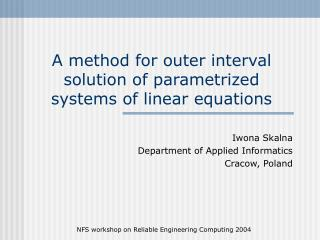DownloadDownload PresentationA method for outer interval solution of parametrized systems of linear equations

# A method for outer interval solution of parametrized systems of linear equations

Télécharger la présentation## A method for outer interval solution of parametrized systems of linear equations

- - - - - - - - - - - - - - - - - - - - - - - - - - - E N D - - - - - - - - - - - - - - - - - - - - - - - - - - -
##### Presentation Transcript

1. A method for outer interval solution of parametrized systems of linear equations Iwona Skalna Department of Applied Informatics Cracow, Poland NFS workshop on Reliable Engineering Computing 2004

2. Parametrized systems Consider a family of linear algeraic systems of the following type where and NFS workshop on Reliable Engineering Computing 2004

3. Solution set This family of linear algebraic systems is usually written in a compact form and is called parametrized system of linear interval equations. The solution set of such system is defined as NFS workshop on Reliable Engineering Computing 2004

4. New method Presented here method is based on the following inclusion where  denotes an interval hull, NFS workshop on Reliable Engineering Computing 2004

5. Algorithm NFS workshop on Reliable Engineering Computing 2004

6. Results In this presentation there is only one example shown, however in the paper some other examples may be found. The results produced by the described method are compared with the results of the method called Random Sampling of Parameter Intervals which gives an inner enclosure of the solution set. NFS workshop on Reliable Engineering Computing 2004

7. Example – plane truss y 30kN 30kN 30kN (0, 9) (4, 9) (8, 9) (12, 9) (16, 9) 1 4 2 3 5 8 6 7 9 (16, 6) (4, 6) (8, 6) (12, 6) 10 11 (16, 3) (12, 3) 12 (16, 0) x NFS workshop on Reliable Engineering Computing 2004

8. Example - description The exemplary plane truss structure is subjected to downward forces of 30 [kN] at nodes 2, 3, 4. All the bar elements have the same Young’s modulus E=7e10 [Pa], and the same cross-section area A=0.003 [m2]. The lengths (in meters) of the bars are shown in the figure. Now the stiffness of some of the bars (denoted in the figure with thick red lines) is assumed to be uncertain by 5%. NFS workshop on Reliable Engineering Computing 2004

9. Results – x coordinates NFS workshop on Reliable Engineering Computing 2004

10. Results – y coordinates NFS workshop on Reliable Engineering Computing 2004

11. Conclusions The problem of solving parametrized systems of linearinterval equations is very important in practicalapplications. Well known classical methods (e.g. Gauss Elimination) fail since they computeenclosure forthe solution set of nonparametrized systems which is generally muchlargerthen solution set of the parametrized ones. A direct method for solvingparaterized systems of linear interval equationswas proposed and checked to be usefullin structuremechanics. The method produced tightenclosure for the solutions set of parametrizedsystemsfor exemplary truss structures. NFS workshop on Reliable Engineering Computing 2004